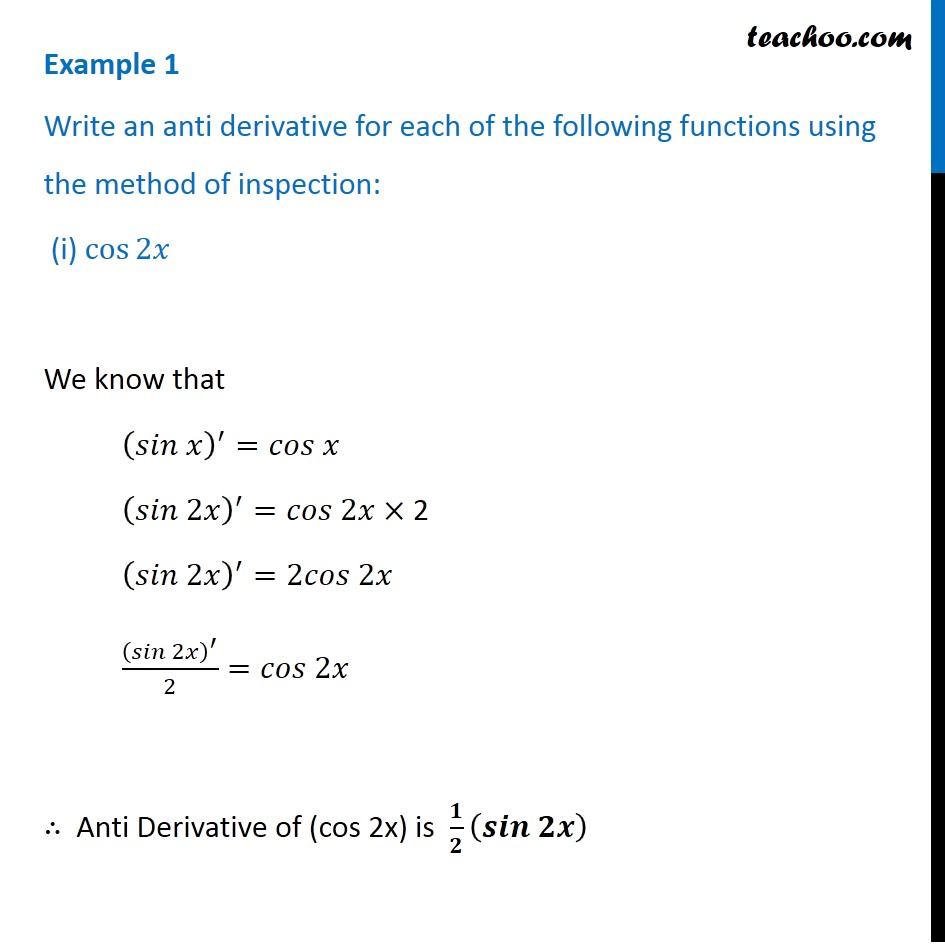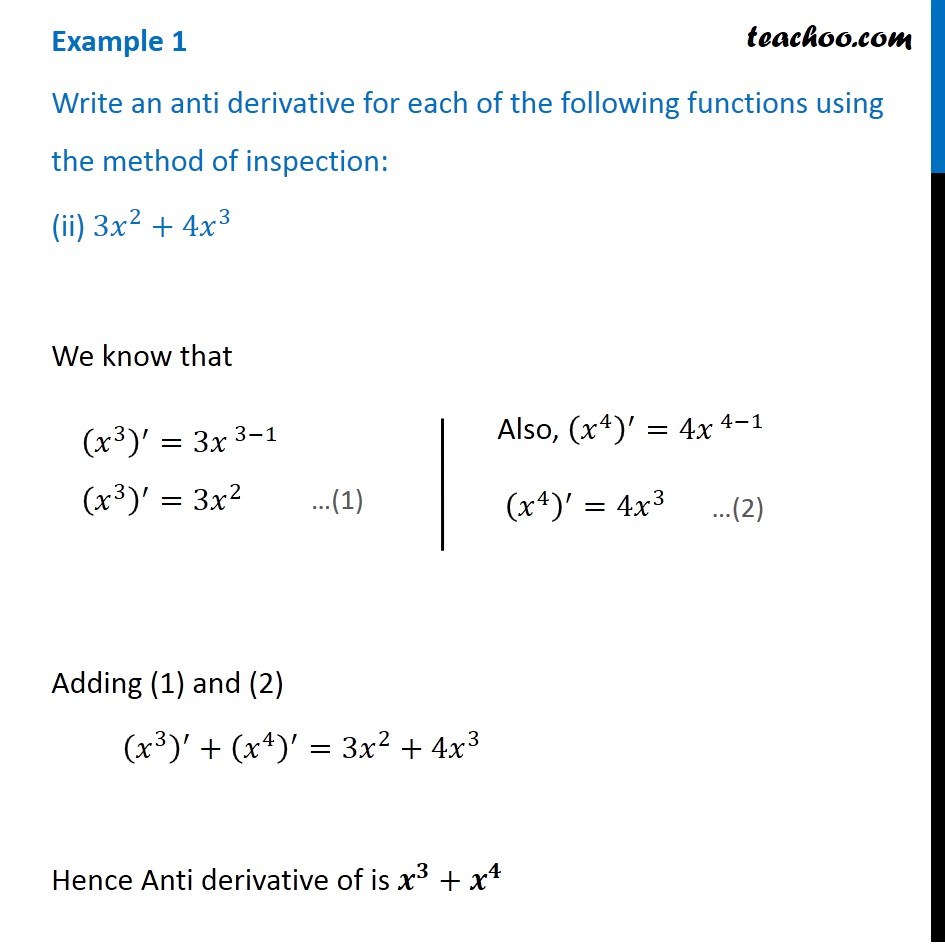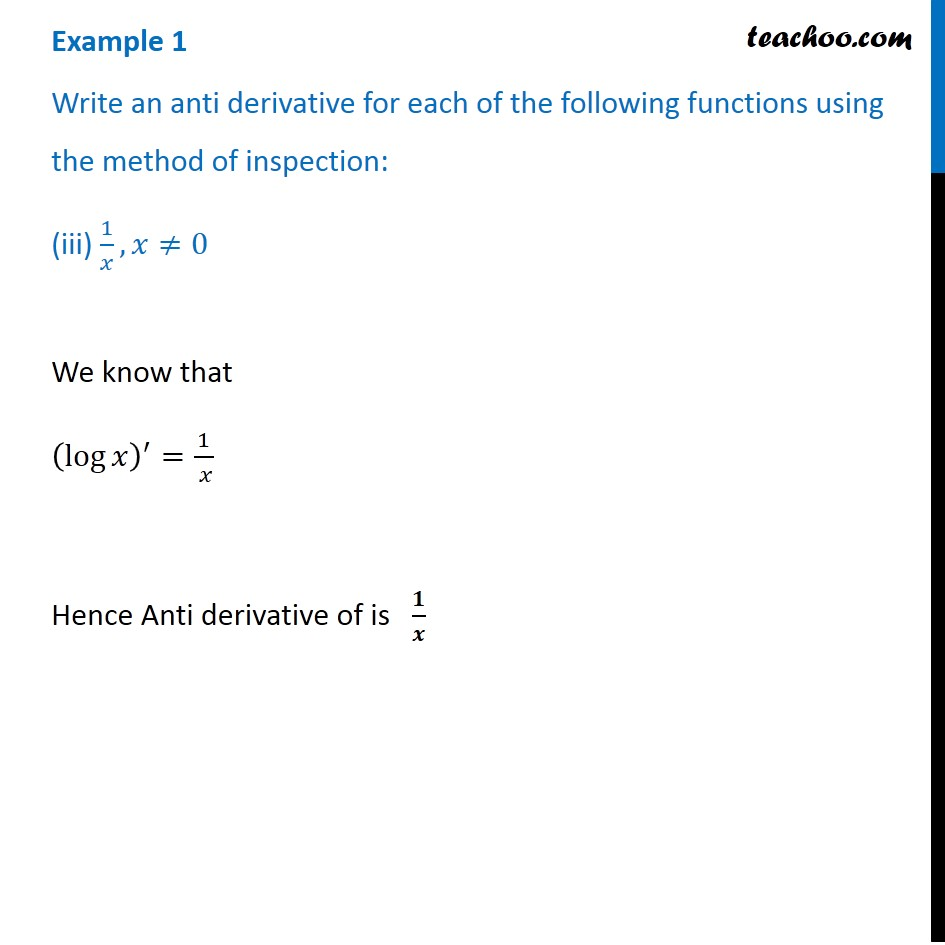1. Chapter 7 Class 12 Integrals
2. Serial order wise
3. Examples

Transcript

Example 1 Write an anti derivative for each of the following functions using the method of inspection: (i) cos⁡2𝑥 We know that (𝑠𝑖𝑛 𝑥)^′=𝑐𝑜𝑠 𝑥 (𝑠𝑖𝑛 2𝑥)^′=𝑐𝑜𝑠 2𝑥× 2 (𝑠𝑖𝑛 2𝑥)^′=2𝑐𝑜𝑠 2𝑥 (𝑠𝑖𝑛 2𝑥)^′/2=𝑐𝑜𝑠 2𝑥 ∴ Anti Derivative of (cos 2x) is 𝟏/𝟐 (𝒔𝒊𝒏 𝟐𝒙) Example 1 Write an anti derivative for each of the following functions using the method of inspection: (ii) 3𝑥^2+4𝑥^3 We know that Adding (1) and (2) (𝑥^3 )^′+(𝑥^4 )^′=3𝑥^2+4𝑥^3 Hence Anti derivative of is 𝒙^𝟑+𝒙^𝟒 (𝑥^3 )^′=3𝑥^( 3−1) (𝑥^3 )^′=3𝑥^2 Also, (𝑥^4 )^′=4𝑥^( 4−1) (𝑥^4 )^′=4𝑥^3 Example 1 Write an anti derivative for each of the following functions using the method of inspection: (iii) 1/𝑥, 𝑥≠0 We know that (log⁡𝑥 )^′=1/( 𝑥) Hence Anti derivative of is 𝟏/𝒙

Examples

Chapter 7 Class 12 Integrals
Serial order wise

About the AuthorDavneet Singh
Davneet Singh is a graduate from Indian Institute of Technology, Kanpur. He has been teaching from the past 10 years. He provides courses for Maths and Science at Teachoo.